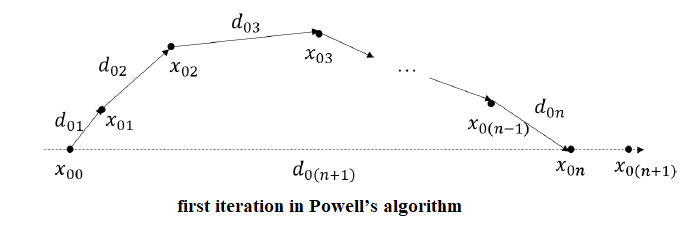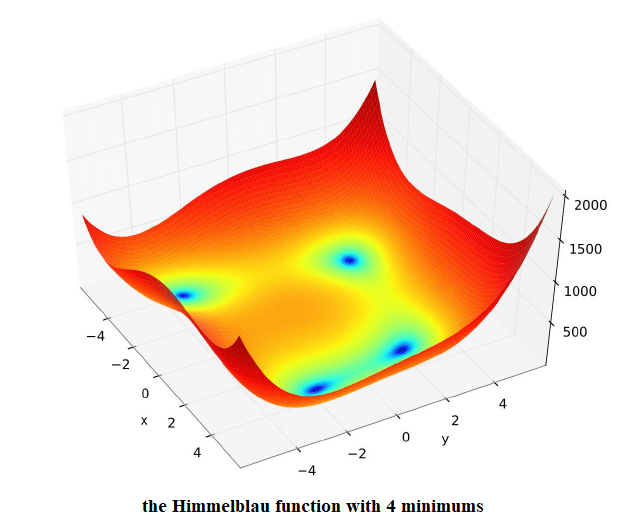The successive search directions in the steepest-descent methods may or may not relate to each other. And also they are determined entirely by the local properties of the bojective functions, the gradient and the Hessian. On the other hand, we see a very strict mathematical relationship between successive search methods and the conjugate direction methods. Conjugate methods were developed for solving quadratic optimization problem with the following objective function$\displaystyle minx^Tb+\frac{1}{2}x^T HX$, where H is an$n*n$ symmetric positive definite matrix for a n-variable function.

For a quadratic problem, the search will converge to a minimum in a finite number of iterations. The conjugate methods have since been used to solve more general optimization problems.

## Conjugate Directions

For a symmetric matrix H, a finite set of vectors${d_0,d_1,...,d_k}$ are said to be conjugate with respect to H or H-orthogonal if$d^T_iQd_j=0$ for all$i\neq j$.If H is positive definite, then the vectors are linearly indeoendent. If H=I, then the vectors are orthogonal.

For the above quadratic minimization problem and when H is positive definite, using simple Calculuc we can show that the solution is unique and can be computed analytically by solving a syasyetm of linear equations,$Hx^*=b$.

Since the set of conjugate vectors are linearly independent, they span the n-dimensional space$E^n,\, x^*$ an be written s a linear combination of those vectors.$x^*=\alpha_0d_0+....+\alpha_{n-1}d_{n-1}$

Now multiplying by H on both sides and taking the scalar product with$d_i$ gives,$\displaystyle \alpha_i-\frac{d^T_i Hx^*}{d^T_i H d_i}=-\frac{d^T_i b}{d^T_i H d_i}$

or we can also write as,$\displaystyle x^*=\sum^{n-1}_{i=0}\frac{d^T_i b}{d^T_i H d_i}d_i$

This expansion f$x^*$ can be considered as a sum or an n-step iterative process of adding$\alpha_i d_i$.

### Conjugate Direction Theorem

Let$\{d_i\}^{n-i}_{i=0}$ be a set of nonzero H-orthogonal vectors. For any$x_0 \epsilon E^n$ the sequence$\{x_i\}$ generated according to$x_{k+1}=x_k+\alpha_kd_k,\, k\geq 0$

with$\displaystyle a_k=-\frac{g^T_k d_k}{d^T_k H d_k}$

and$g_k=b+Hx_k$ convertges to the unique olution$x^*$ of$HX=b$ after n steps, that is$x_n=x^*$.

Using the above theorem and a number of ways to generate conjugate vectors, a number of conjugate-direction methods can be devised. We also need to extend the theorem to solve non-quadratic problems. The conjugate-directiopn method is compatible with the steepest-descent framework. They only differ by the direction of vectors$d_k$ and the magnitude$a_k$ is computed consequently. We will use here theconjugative-direction methods instead of the steepest-descent methods. The idea of iteratively adding an increment$a_k d_k$ to generate$x_{k+1}$ is the same.

In this method, the new direction is a weihted sum of the last direction and the negative of the gradient.$d_0=-g_0=-(b+Hx_0)$$d_k=-g_k+ \beta_k d_{k-1}$$g_k=b+Hx_k$$\displaystyle \beta_k=\frac{g_k^T Hd_k}{d^T_k Hd_k}$$\displaystyle \alpha_k=-\frac{g^T_k Hd_k}{d^T_k Hd_k}$

The advantages of this method are:

1. The gradient is finite and is linearly independent of all previous direction vectors, except when the solution is found.

2. It is relatively easy to compute a new direction vector.

3. There is no line search.

4. It converges in n steps for quadratic problems.

5. The first direction is the same as in steepest-descent method,$d_0=-g_0$, and it gives a first good reduction.

6. There is no inversion of Hessian.

1. Hessian must be supplied, stored and manipulated.

2. Convergence is not guranteed for on-quadratic problems. In fact, if the initial point is far from a  solution, the search may wander in some sub-optimal area because unreliable directions build up over iterations. As we will see from the code example later on, the conjugate-gradient method takes the biggest number of iterations to converge for the Himmelblau function.

## Fletcher Reeves Method

The Fletcher Reeves Method is a variant of the onjugate-gradient method. The visibel difference is that$\alpha_k$ is computed using a line search by minimizing$f(X+\alpha_k d_k)$ and here$d_k$ is a conjugate direction with respect to$\{d_0,d_1,...,d_k\}$ rather than in the steepest-descent method or Newton methods. Also, the algorithim is similar to that of the conjugate-gradient method but here it requires more computations because of the line search involved which is a setback when compared with the conjugate-gradient method. However, there are two major advantages of this particular method:

1. The modification works better for minimization of non-quadratic functions because  larger reduction can be achieved in$f(x)$ along$d_k$ at points outside the neighbourhood of a solution.

2. This algorithim does not need the Hessian.## Powell Method

Powel’s algorithim generates a series of algorithim of conjugate directions using a line search.

Theorem of Generation of Conjugate Directions in Powell’s Method:

Let$x^*_a$ and$x^*_b$ be the minimizers obtained if the convex quadratic function$\displaystyle f(x)=x^T+\frac{1}{2}x^THx$

is minimized with respect to a$\alpha$ on the lines$d_a$ and$d_b$$x=x_a+\alpha d_a$

and$x=x_b +\alpha d_b$

If$d_a=d_b$, then the vector$x^*_b-x^*a$ is conjugate with respect to$d_a$.

In this algorithim, the initial point$x_{00}$ and n linearly independent vectors$\{d_0,d_1,...,d_n\}$, taking the coordinate directions are assumed and a series of line searches are performed in each iterations . In the first iteration,$f(x)$ is minimized sequentially in the directions of the vectors starting rom the initial point to the yield point$x_{01},x_{02},...,x_{0n}$. A new vector is then generatd and is given by$d_{0(n+1)}=x_{0n}-x_{00}$.$f(x)$ is then minimized in the direction of this new vector to yield the new point$x_{0(n+1)}$. The vectors are updated by setting$d_{11}=d_{02}$$d_{12}=d_{03}$

…….$d_{1(n-1)}=d_{0n}$$d_{1n}=d_{0(n+1)}$

The same process is repeated in the second iteration and starts from the point$x_{10}=x_{0(n+1)}$.$f(x)$ is then minimized sequentially in the direction of the vectors$\{d_1,d_2,....,d_{1n}\}$ to yield the points$x_{11},x_{12},...,x_{1n}$. Then the new vector is generated as$d_{1(n+1)}=x_{1n}-x_{10}$.

Proceeding in the same way, each new iteration will increase one conjugate vector and remove one other, similar toa substitution. Since each iteration requires$(n+1)$ line searches, Powell’s method performs$n(n+1)$ line searches in n iterations.

The advantages of the Powell’s method is that it does not supply, store or manipulate the Hessian nor does it needs the gradient. However, it may not be able to generate a linearly independent set of vectors spanning$E^n$ and it is not necessary that the algorithgim may converge to a solution.## Zangwill Method

Zangwill’s algorithim is an improved version of the Powell’s algorithim. It generates a set of conjugate set of vectors that are always linearly independent. Therefore, it eliminates the disadvantage in the Powell’s algorithim.

The modifications in this method are that the first set of coordinates are chosen and that their determinant is 1. Second, the new set of vectors is normalised to unit length. Third, the direction substation must maintain the determinant of the matrix of the direction vectors is positive and still less than or equal to 1. The last item ensures that the direction vectors re always linearly independent.The following code minimizes the Himmelblau function$f(x_1,x_2)=(x_1^2+x_2-11)^2+(x_1+x_2^2-7)^2$

				
//The Himmelblau function:
// f(x) = (x1^2 + x2 - 11)^2 + (x1 + x2^2 - 7)^2

val f: RealScalarFunction f= object : new RealScalarFunction() {
override fun Double evaluate(Vector x) {
double x1 = x.get(1),
double x2 = x.get(2),

double result = pow(x1 * x1 + x2 - 11, 2),
result += 12 * pow(x1 + x2 * x2 - 7, 2),
return result
}
override public int dimensionofDomain() {
return 2
}
override public int dimensionofRange() {
return 1
}
}

val g: RealVectorFunction g = object : new RealVectorFunction() {
override fun evaluate(Vector x): {
double x1 = x.get(1),
double x2 = x.get(2),

double w1 = x1 * x1 + x2 - 11,
double w2 = x1 + x2 * x2 -7,

double[] result = new double,
result = 4 * w1 * x1 + 2 * w2,
result = 2 * w1 + 4 * w2 * x2,
return new DenseVector (result)
}
override public int dimensionofDomain() {
return 2
}
override public int dimensionofRange() {
return 2
}
}

val H: RntoMatrix H = object : new RntoMatrix() {
override Matrix evaluate(Vector x): {
double x1 = x.get(1),
double x2 = x.get(2),

Matrix result = new DenseMatrix(2, 2),
result.set(1, 1, 12 * x1 * x1 + 4 * x2 - 42),
result.set(1, 2, 4 * (x1 + x2)),
result.set(2, 1, result.get(1, 2)),
result.set(2, 2, 4 * x1 + 12 * x2 * x2 - 26),
return result
}

override public int dimensionofDomain() {
return 2
}
override public int dimensionofRange() {
return 1
}
}

val problem: C2OptimProblem = new C2OptimProblemImpl(f, g, H)
1e-16,
40)
val xmin1: soln1.search(new DenseVector(new double[]{6, 6}))
val fmin1= f.evaluate(xmin1)
System.out.println(String.format("f(%s) = %.16f", xmin1.toString(), fmin1))

val solver : ConjugateGradientMinimizer FletcherReevesMinimizer = new FletcherReevesMinimizer(
1e-16,
20)
val soln2: FletcherReevesMinimizer.solve(problem)
val xmin2: soln2.search(new DenseVector(new double[]{6, 6}))
val fmin2= f.evaluate(xmin2)
System.out.println(String.format("f(%s) = %.16f", xmin2.toString(), fmin2))

val solver : ConjugateGradientMinimizer PowellMinimizer = new PowellMinimizer(
1e-16,
20)
val soln3: PowellMinimizer.solve(problem)
val xmin3: soln3.search(new DenseVector(new double[]{6, 6}))
val fmin3= f.evaluate(xmin3)
System.out.println(String.format("f(%s) = %.16f", xmin3.toString(), fmin3))

val solver : ConjugateGradientMinimizer ZangwillMinimizer = new ZangwillMinimizer(
1e-16,
1e-16,
20)
val soln4: ZangwillMinimizer.solve(problem)
val xmin4: soln4.search(new DenseVector(new double[]{6, 6}))
val fmin4= f.evaluate(xmin4)
System.out.println(String.format("f(%s) = %.16f", xmin4.toString(), fmin4))



The output is:

				
f([3.000000, 2.000000]) = 0.0000000000013999
f([3.000002, 1.999998]) = 0.0000000001278725
f([-2.805114, 3.131310]) = 0.0000000007791914
f([-2.805117, 3.131311]) = 0.0000000001359077



The two minimums found are (3,2) and (-2.805118, 3.131312)